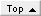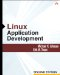# Section 7.4. Investigating Memory Errors with Valgrind

### 7.4. Investigating Memory Errors with Valgrind

Valgrind is an Intel x86-specific tool that emulates an x86-class CPU to watch all memory accesses directly and analyze data flow (for example, it can recognize reads of uninitialized memory, but it also recognizes that moving one uninitialized value into another location that is never read does not actually constitute an uninitialized read). It has many other capabilities, including investigating cache use and looking for race conditions in threaded programs, and in fact has a general facility for adding more capabilities based on its CPU emulator. However, for our purposes, we merely briefly introduce its aggressive memory error checking, which is its default behavior.

 Available from http://valgrind.kde.org/

Valgrind does not require that a program be recompiled, although its analysis, like all debugging tools, is enhanced by compiling the program with debugging information included.

` \$ valgrind ./broken ==30882== Memcheck, a.k.a. Valgrind, a memory error detector for x86-linux. ==30882== Copyright (C) 2002-2003, and GNU GPL'd, by Julian Seward. ==30882== Using valgrind-2.0.0, a program supervision framework for x86-linux. ==30882== Copyright (C) 2000-2003, and GNU GPL'd, by Julian Seward. ==30882== Estimated CPU clock rate is 1547 MHz ==30882== For more details, rerun with: -v ==30882== ==30882== Invalid write of size 1 ==30882==    at 0xC030DB: strcpy (mac_replace_strmem.c:174) ==30882==    by 0x8048409: broken (broken.c:15) ==30882==    by 0x804851F: main (broken.c:47) ==30882==    by 0x802BAE: __libc_start_main (in /lib/libc-2.3.2.so) ==30882==    Address 0x650F029 is 0 bytes after a block of size 5 alloc'd ==30882==    at 0xC0C28B: malloc (vg_replace_malloc.c:153) ==30882==    by 0x80483F3: broken (broken.c:14) ==30882==    by 0x804851F: main (broken.c:47) ==30882==    by 0x802BAE: __libc_start_main (in /lib/libc-2.3.2.so) ==30882== ==30882== Conditional jump or move depends on uninitialised value(s) ==30882==    at 0x863D8E: __GI_strlen (in /lib/libc-2.3.2.so) ==30882==    by 0x83BC31: _IO_printf (in /lib/libc-2.3.2.so) ==30882==    by 0x804841C: broken (broken.c:16) ==30882==    by 0x804851F: main (broken.c:47) 1: 12345 ==30882== ==30882== Invalid write of size 1 ==30882==    at 0xC030D0: strcpy (mac_replace_strmem.c:173) ==30882==    by 0x804844D: broken (broken.c:21) ==30882==    by 0x804851F: main (broken.c:47) ==30882==    by 0x802BAE: __libc_start_main (in /lib/libc-2.3.2.so) ==30882==    Address 0x650F061 is 0 bytes after a block of size 5 alloc'd ==30882==    at 0xC0C28B: malloc (vg_replace_malloc.c:153) ==30882==    by 0x8048437: broken (broken.c:20) ==30882==    by 0x804851F: main (broken.c:47) ==30882==    by 0x802BAE: __libc_start_main (in /lib/libc-2.3.2.so) ==30882== ==30882== Invalid write of size 1 ==30882==    at 0xC030DB: strcpy (mac_replace_strmem.c:174) ==30882==    by 0x804844D: broken (broken.c:21) ==30882==    by 0x804851F: main (broken.c:47) ==30882==    by 0x802BAE: __libc_start_main (in /lib/libc-2.3.2.so) ==30882==    Address 0x650F064 is 3 bytes after a block of size 5 alloc'd ==30882==    at 0xC0C28B: malloc (vg_replace_malloc.c:153) ==30882==    by 0x8048437: broken (broken.c:20) ==30882==    by 0x804851F: main (broken.c:47) ==30882==    by 0x802BAE: __libc_start_main (in /lib/libc-2.3.2.so) ==30882== ==30882== Invalid read of size 4 ==30882==    at 0x863D50: __GI_strlen (in /lib/libc-2.3.2.so) ==30882==    by 0x83BC31: _IO_printf (in /lib/libc-2.3.2.so) ==30882==    by 0x8048460: broken (broken.c:22) ==30882==    by 0x804851F: main (broken.c:47) ==30882==    Address 0x650F064 is 3 bytes after a block of size 5 alloc'd ==30882==    at 0xC0C28B: malloc (vg_replace_malloc.c:153) ==30882==    by 0x8048437: broken (broken.c:20) ==30882==    by 0x804851F: main (broken.c:47) ==30882==    by 0x802BAE: __libc_start_main (in /lib/libc-2.3.2.so) ==30882== ==30882== Invalid read of size 1 ==30882==    at 0x857A21: _IO_file_xsputn@@GLIBC_2.1 (in /lib/libc-2.3.2.so) ==30882==    by 0x835309: _IO_vfprintf_internal (in /lib/libc-2.3.2.so) ==30882==    by 0x83BC31: _IO_printf (in /lib/libc-2.3.2.so) ==30882==    by 0x8048460: broken (broken.c:22) ==30882==    Address 0x650F063 is 2 bytes after a block of size 5 alloc'd ==30882==    at 0xC0C28B: malloc (vg_replace_malloc.c:153) ==30882==    by 0x8048437: broken (broken.c:20) ==30882==    by 0x804851F: main (broken.c:47) ==30882==    by 0x802BAE: __libc_start_main (in /lib/libc-2.3.2.so) ==30882== ==30882== Invalid read of size 1 ==30882==    at 0x857910: _IO_file_xsputn@@GLIBC_2.1 (in /lib/libc-2.3.2.so) ==30882==    by 0x835309: _IO_vfprintf_internal (in /lib/libc-2.3.2.so) ==30882==    by 0x83BC31: _IO_printf (in /lib/libc-2.3.2.so) ==30882==    by 0x8048460: broken (broken.c:22) ==30882==    Address 0x650F061 is 0 bytes after a block of size 5 alloc'd ==30882==    at 0xC0C28B: malloc (vg_replace_malloc.c:153) ==30882==    by 0x8048437: broken (broken.c:20) ==30882==    by 0x804851F: main (broken.c:47) ==30882==    by 0x802BAE: __libc_start_main (in /lib/libc-2.3.2.so) 2: 12345678 ==30882== ==30882== Invalid write of size 1 ==30882==    at 0x8048468: broken (broken.c:25) ==30882==    by 0x804851F: main (broken.c:47) ==30882==    by 0x802BAE: __libc_start_main (in /lib/libc-2.3.2.so) ==30882==    by 0x8048354: (within /usr/src/d/lad2/code/broken) ==30882==    Address 0x650F05B is 1 bytes before a block of size 5 alloc'd ==30882==    at 0xC0C28B: malloc (vg_replace_malloc.c:153) ==30882==    by 0x8048437: broken (broken.c:20) ==30882==    by 0x804851F: main (broken.c:47) ==30882==    by 0x802BAE: __libc_start_main (in /lib/libc-2.3.2.so) ==30882== ==30882== Invalid read of size 4 ==30882==    at 0x863D50: __GI_strlen (in /lib/libc-2.3.2.so) ==30882==    by 0x83BC31: _IO_printf (in /lib/libc-2.3.2.so) ==30882==    by 0x804847A: broken (broken.c:26) ==30882==    by 0x804851F: main (broken.c:47) ==30882==    Address 0x650F064 is 3 bytes after a block of size 5 alloc'd ==30882==    at 0xC0C28B: malloc (vg_replace_malloc.c:153) ==30882==    by 0x8048437: broken (broken.c:20) ==30882==    by 0x804851F: main (broken.c:47) ==30882==    by 0x802BAE: __libc_start_main (in /lib/libc-2.3.2.so) ==30882== ==30882== Invalid read of size 1 ==30882==    at 0x857A21: _IO_file_xsputn@@GLIBC_2.1 (in /lib/libc-2.3.2.so) ==30882==    by 0x835309: _IO_vfprintf_internal (in /lib/libc-2.3.2.so) ==30882==    by 0x83BC31: _IO_printf (in /lib/libc-2.3.2.so) ==30882==    by 0x804847A: broken (broken.c:26) ==30882==    Address 0x650F063 is 2 bytes after a block of size 5 alloc'd ==30882==    at 0xC0C28B: malloc (vg_replace_malloc.c:153) ==30882==    by 0x8048437: broken (broken.c:20) ==30882==    by 0x804851F: main (broken.c:47) ==30882==    by 0x802BAE: __libc_start_main (in /lib/libc-2.3.2.so) ==30882== ==30882== Invalid read of size 1 ==30882==    at 0x857910: _IO_file_xsputn@@GLIBC_2.1 (in /lib/libc-2.3.2.so) ==30882==    by 0x835309: _IO_vfprintf_internal (in /lib/libc-2.3.2.so) ==30882==    by 0x83BC31: _IO_printf (in /lib/libc-2.3.2.so) ==30882==    by 0x804847A: broken (broken.c:26) ==30882==    Address 0x650F061 is 0 bytes after a block of size 5 alloc'd ==30882==    at 0xC0C28B: malloc (vg_replace_malloc.c:153) ==30882==    by 0x8048437: broken (broken.c:20) ==30882==    by 0x804851F: main (broken.c:47) ==30882==    by 0x802BAE: __libc_start_main (in /lib/libc-2.3.2.so) 3: 12345678 4: 12345 ==30882== ==30882== Invalid write of size 1 ==30882==    at 0x80484A6: broken (broken.c:32) ==30882==    by 0x804851F: main (broken.c:47) ==30882==    by 0x802BAE: _ _libc_start_main (in /lib/libc-2.3.2.so) ==30882==    by 0x8048354: (within /usr/src/d/lad2/code/broken) ==30882==    Address 0xBFF2D0FF is just below %esp.  Possibly a bug in GCC/G++ ==30882==    v 2.96 or 3.0.x.  To suppress, use: --workaround-gcc296-bugs=yes 5: 12345 6: 12345 7: 12345 ==30882== ==30882== ERROR SUMMARY: 22 errors from 12 contexts (suppressed: 0 from 0) ==30882== malloc/free: in use at exit: 5 bytes in 1 blocks. ==30882== malloc/free: 2 allocs, 1 frees, 10 bytes allocated. ==30882== For a detailed leak analysis,  rerun with: --leak-check=yes ==30882== For counts of detected errors, rerun with: -v `

Note that Valgrind found everything but the global overflow and underrun, and it pinpointed the errors more specifically than any other tool we have described here.

One option allows you to turn on an aggressive form of leak checking in which the program is searched to determine for each memory allocation whether any accessible pointers still hold a reference to that memory. This is more accurate than simply asking whether memory has been free() ed because it is reasonably common to allocate some memory that is held for the lifetime of the program, and not to free() it because it returns to the operating system when the program exits anyway.

` \$ valgrind --leak-check=yes ./broken ... ==2292== searching for pointers to 1 not-freed blocks. ==2292== checked 5318724 bytes. ==2292== ==2292== 5 bytes in 1 blocks are definitely lost in loss record 1 of 1 ==2292==    at 0xEC528B: malloc (vg_replace_malloc.c:153) ==2292==    by 0x8048437: broken (broken.c:20) ==2292==    by 0x804851F: main (broken.c:47) ==2292==    by 0x126BAE: __libc_start_main (in /lib/libc-2.3.2.so) ==2292== ==2292== LEAK SUMMARY: ==2292==    definitely lost: 5 bytes in 1 blocks. ==2292==    possibly lost:   0 bytes in 0 blocks. ==2292==    still reachable: 0 bytes in 0 blocks. ==2292==         suppressed: 0 bytes in 0 blocks. ==2292== Reachable blocks (those to which a pointer was found) are not shown. ==2292== To see them, rerun with: --show-reachable=yes `

Valgrind includes fairly detailed information on its capabilities, called skins, and has many command-line options for modifying its behavior.

Because Valgrind uses a CPU emulator, it runs many times slower than a program running natively on the system. Exactly how much slower depends on the program, but Valgrind is intended to be at least usable for running interactive programs.

There are occasional subtle issues that can confuse Valgrind when you compile with high levels of optimization. If you get a report of a memory error that does not seem to make sense, try compiling with -0 rather than -02 (or higher) and see if the report changes.Linux Application Development (paperback) (2nd Edition)
ISBN: 0321563220
EAN: 2147483647
Year: 2003
Pages: 168

Similar book on Amazon